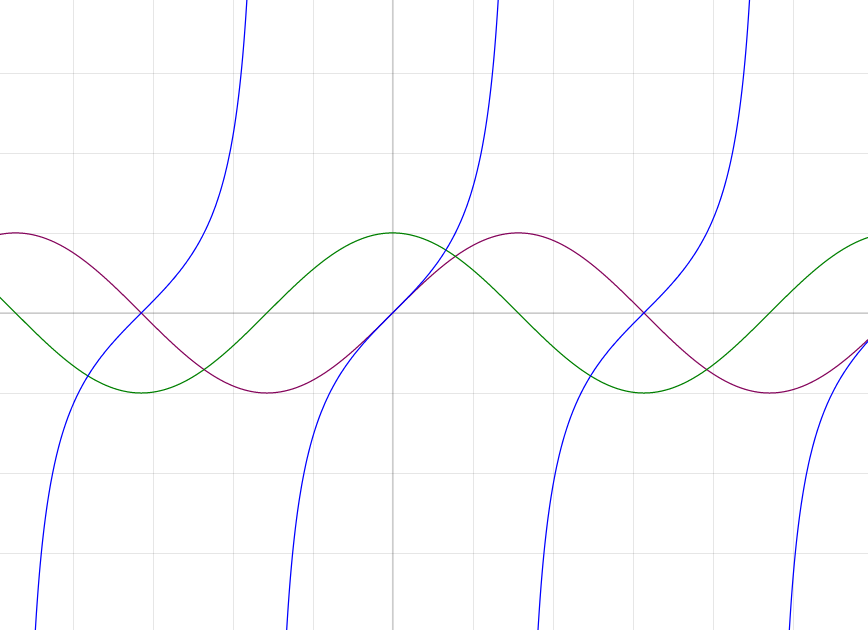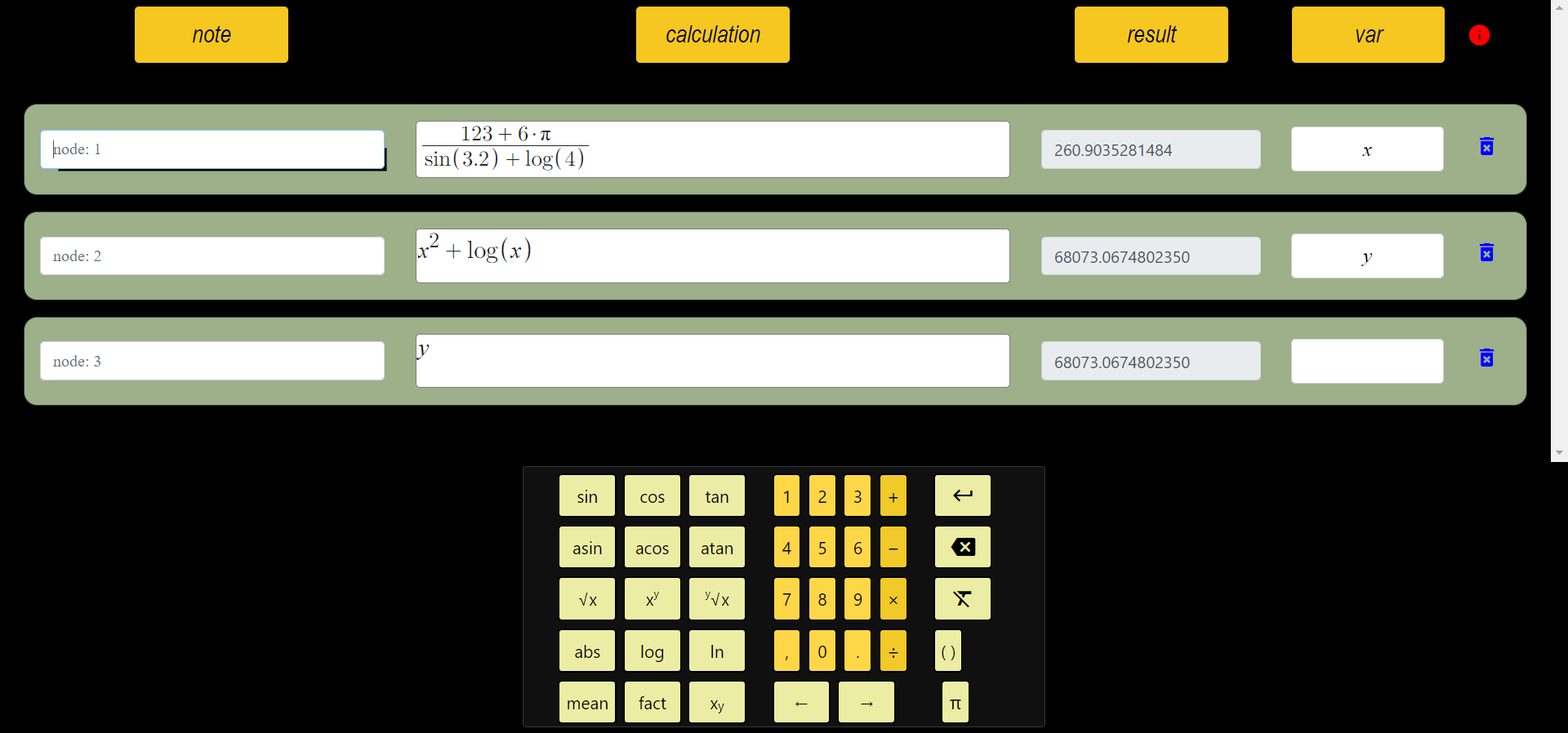# Trigonometry - Triangle Mathematics/Geometry

Trigonometry is a branch of mathematics that studies triangles. Trigonometry is a field of mathematics that studies the properties of triangles using the side lengths and angles of triangles. Trigonometric functions help compute the properties of triangles using angles and side lengths in triangles. These functions are called sine, cosine, and tangent.

An important application of trigonometry is when working in coordinate systems. For example, if the coordinates of a point are known, trigonometry is used to figure out how we can find that point on a coordinate line. In addition, trigonometry plays an important role in physics, engineering and other fields. For example, in physics, trigonometry is used to solve Newton's laws of motion.

Trigonometry is an important subject in mathematics and is taught in many university programs. Graphs of trigonometric functions are similar to each other. Trigonometry helps students understand the importance of math topics such as linear algebra, triangles, and plane geometry. Trigonometry also plays an important role in solving many mathematical problems and is an important tool in many fields.

## Trigonometric Functions

Trigonometric functions convert the value of an angle in degrees to a ratio. There are seven of these functions, sine, cosine, tangent, cosine, sine inverse, cosine inverse and tangent inverse. These functions are named according to their trigonometric properties.

• The sine function returns the height of the response of an angle. For example, the value of sin(30) would be 0.5.
• The cosine function returns the length of the response of an angle. For example, cos(45) would be 0.7071067811865475.
• The tangent function returns the ratio of the sine and cosine functions. For example, the value tan(45) would be 1.
• The cosine function is the inverse of the cosine function and is subtracted from 1. For example, cos(60) would be 0.5.
• The sine inverse function is the inverse of the sine function and is converted to radians. For example, the sine inverse(0.5) would be 30.
• The cosine inverse function is the inverse of the cosine function and is converted to radians. For example, the cosine inverse(0.7071067811865475) would be 45.
• The tangent inverse function is the inverse of the tangent function and is converted to radians. For example, the tangent inverse(1) value would be 45.

## Sine Function

In mathematics, the sine function y is commonly used and is: y = sin(x)This gives the sine of y relative to the value of x radians. The sine function is used in a variety of math and physics applications. For example, trigonometric functions are often used in geometry and engineering. The sine function can also be used to find the coordinates of a point in a pattern.

There is also the arcsine function, which is the inverse function of the sine function. This function takes the inverse of the sine function and converts a radian value to a sine value. For example, arcsin(0.5) = 30 degrees.

The sine function also gives the height of a regular wave function and is therefore often used in the modeling of sound and electromagnetic waves.

The sine function is also important in the field of signal processing. For example, you can use the sine function to find the frequency of a signal or to reproduce a signal. It also helps to understand the relationships between signals. For example, a variation of the sine function on a signal indicates a frequency variation of that signal.

## Trigonometric Transformation Formulas

Trigonometric transformation formulas are formulas that allow the conversion of trigonometric functions to each other. They are especially used when studying trigonometric functions in space. These formulas are generally used to convert trigonometric functions to each other and facilitate the conversion of trigonometric functions to each other. Below is a list of trigonometric transformation formulas:

• sin(A+B) = sinAcosB+cosAsinB
• sin(A-B) = sinAcosB-cosAsinB
• cos(A+B) = cosAcosB-sinAsinB
• cos(A-B) = cosAcosB+sinAsinB
• tan(A+B) = (tanA+tanB)/(1-tanAtanB)
• tan(A-B) = (tanA-tanB)/(1+tanAtanB)
• sin 2A = 2sinAcosA
• cos 2A = cos^2A-sin^2A = 1-2sin^2A = 2cos^2A-1
• tan 2A = 2tanA/(1-tan^2A)
These graphs of functions show periodic properties.

The use of these formulas facilitates the conversion of trigonometric functions to each other, and these formulas are often used when working in space. For example, if the values of the trigonometric functions of the coordinates of a mass point are known, these formulas are used to convert these values to each other. In addition, the complex equations of these functions Graphics of these functions in 3D space can be drawn.

###### All Articles

id: 6zP0nUQUck